# How to Convert Multiple Rows to Columns in Excel?

Let's assume you already have a list of data with many rows that only has one column in an excel sheet, but you want to convert these data to multiple rows and columns, you can achieve this using an Excel formula.

## Step 1

Let’s consider the following list of multiple rows data and try to convert those data into multiple columns and rows using excel formula.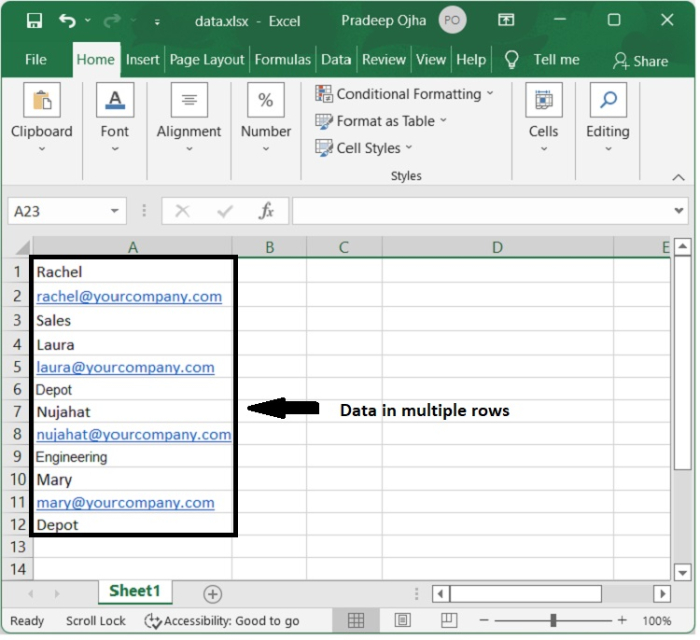## Step 2

Let’s choose an empty cell (C1) in which we will store the first data of the list of data and type the following formula in cell C1 and press Enter.

=OFFSET($A$1,(ROW()-1)*3+INT((COLUMN()-3)),MOD(COLUMN()-3,1))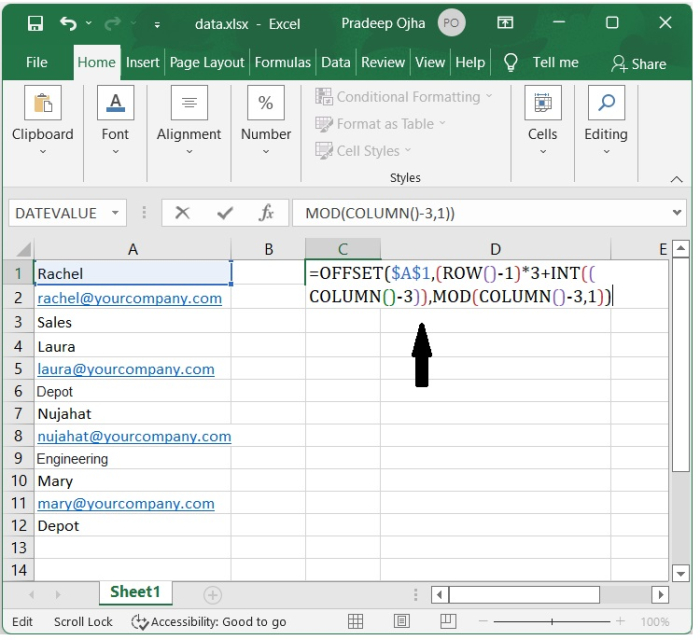## Step 3

Now we get the first-row data of the list data in the cell C1 as shown in the following image.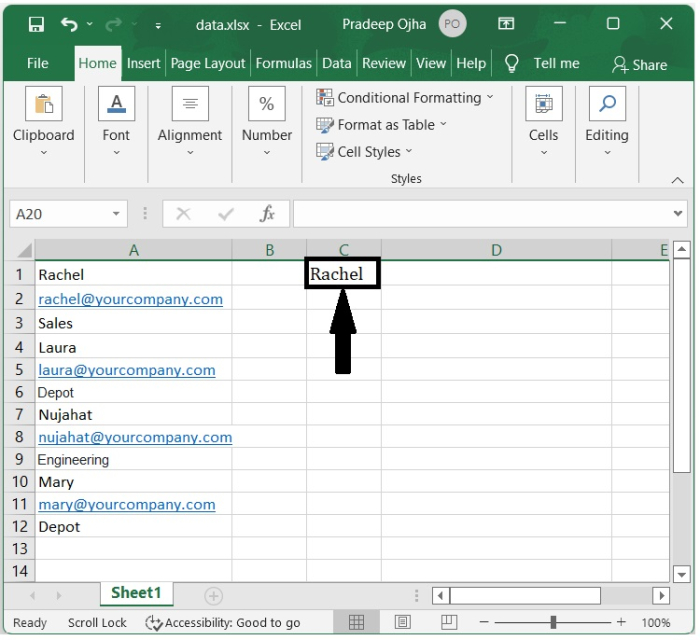## Step 4

To convert all the list data into multiple columns and rows, we have to simply drag the autofill handle from cell C1.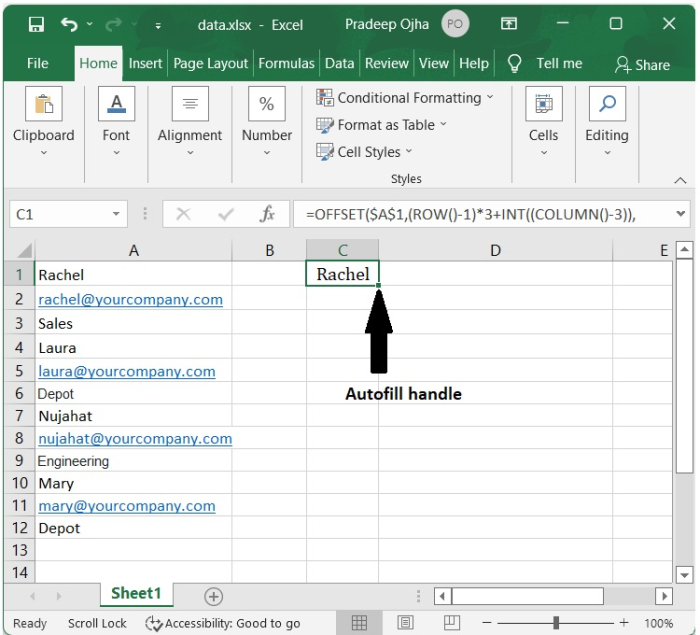## Step 5

Drag the autofill handle from cell C1 to the right cells as our need, followed by dragging the autofill handle downward to the cells till the value zero is displayed. The data is now displayed in multiple rows and columns as shown in the following screenshot.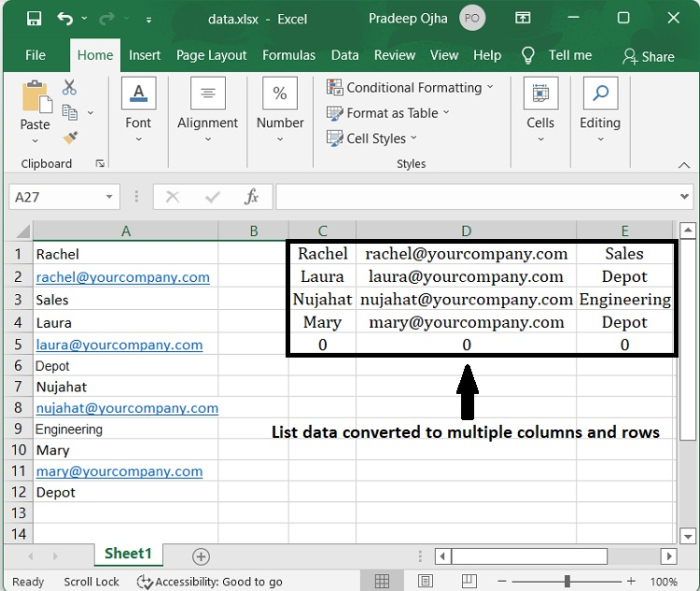The formula we have used above to convert the list data of multiple rows into multiple columns and rows can be interpreted as follows −

OFFSET($A$1,(ROW()- f_row)*rows_in_set+INT((COLUMN()f_col)/col_in_set), MOD(COLUMN()-f_col,col_in_set))

Where,

• f_row − row number of this offset formula.

• f_col − column number of this offset formula.

• rows_in_set − number of rows that make one record of data from the original data.

• col_in_set − number of columns of the original list data.

You can change the values of the above fields in the formula according to your list data of multiple rows.

## Conclusion

In this tutorial, you learned how to convert list data of multiple rows into multiple columns and rows using a worksheet formula.

Updated on: 07-Jul-2022

1K+ Views